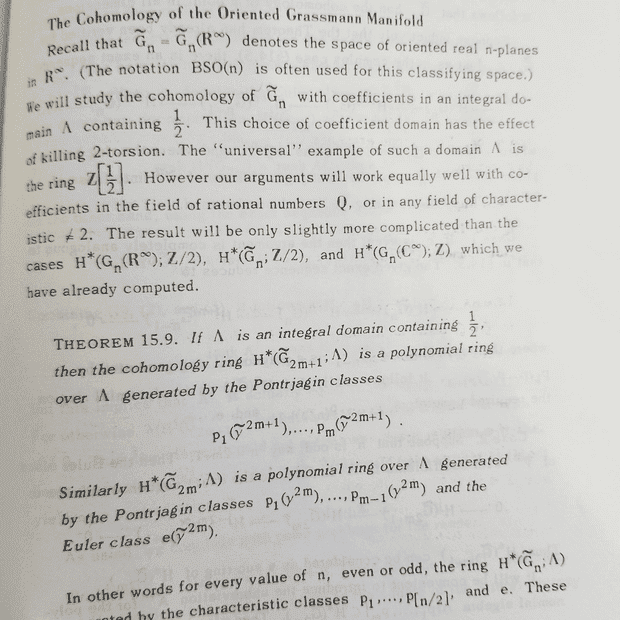# Confused by a theorem in Milnor-Stasheff

• A
• quasar987

#### quasar987

Homework Helper
Gold Member
The k-th Pontrjagin class of a real vector bundle is defined as the 2k-Chern class of the complexified bundle. Therefor, a Pontrjagin class lives in cohomology with integer coefficients. But then the statement of Theorem 15.9 is that if the coefficient ring is taken to be a PID $\Lambda$ containing 1/2 (ex: Z[1/2] or Q, R, C), then the singular cohomology ring of a certain space G is the polynomial ring over $\Lambda$ in the Pontrjagin classes. But what is meant by a Pontrjagin class as an element of $H^*(G,\Lambda)$ ?? Is there a natural map $H^*(G,\mathbb{Z})\rightarrow H^*(G,\Lambda)$ that allows such an identification!?